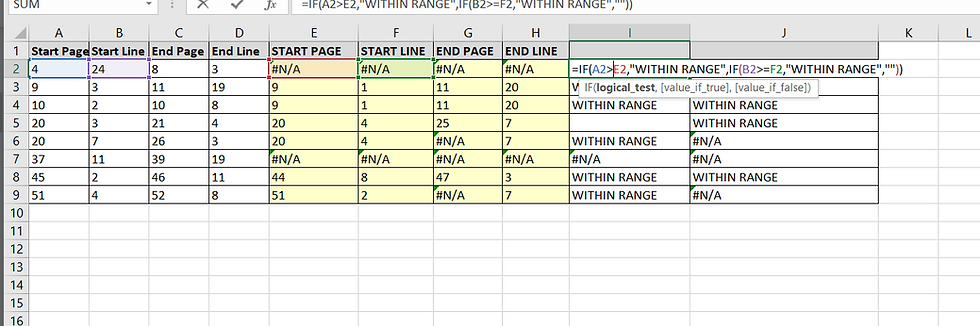top of pageSearch

# checking for overlapping deposition designations

If you have a list of page and line deposition designations and want to see not only where they match marked page and lines from a previous set, but also where they overlap, you can use the Excel spreadsheet that I have posted below.

1. Begin by placing the page and line ranges for your new set of designations in columns A to D.

2. Put the page and line ranges of the designations from a different set that you want to compare the new set against in columns M to P.

3. In column E, enter a LOOKUP formula to find where the page listed in column A falls between the page ranges in columns M and O.It is set to lookup where the value in A falls between the values in column M and O, and then return the value in column M.

4. The formula is repeated in column F, but set to return the line number in column N where the page in A falls between M and O.

5. The LOOKUP formula is then modified in column G to search for where the ending page range in the new set falls with the page ranges of the old set. We can fhus find both where a range in the new set begins within a range from the old set, falls entirely within it, or ends before the range from the old set does.6. Repeat the formula from column G, in column H so that it returns the ending line number from the corresponding range in the old set: =LOOKUP(2,1/((C2>=M:M)*(A2<=O:O)),P:P)

7. In column I a IF . . . THEN formula is used to check if the page in A is greater than the returned range from the old set, and so then must be within the old range, or if the line number at the beginning of the new range is greater or equal than that in the corresponding range from the old set.8. The formula in column J checks where the line number from the new range is less than or equal to that of the corresponding old range, if the page number is not less than the ending page number in the range from the old set.Where a range from the new set falls entirely within a range from the old set, 'WITHIN RANGE' will appear in both column I and J. Where a range from the new set begins within a range from the old set, but continues after it, 'WITHIN RANGE' will only appear in column I.

Where the range from the new set does not begin within a range from the old set, but ends within it, 'WITHIN RANGE' will only appear in column J.Note that this spreadsheet will not show instances where a range from the new set begins before and ends after a range from the old set. To find these instances, swap the old and new ranges.

Overlapping Deposition Designations
.xlsx